Resonance Particle

The following article is from The Great Soviet Encyclopedia (1979). It might be outdated or ideologically biased.

Resonance Particle

(also elementary-particle resonance), the short-lived excited state of strongly interacting elementary particles (hadrons). Unlike other unstable particles, resonance particles decay chiefly as a result of strong interactions; consequently, their lifetimes are between 1022 and 10-24sec. This agrees in order of magnitude with the characteristic nuclear time τnu = Rnu/c ≈ 10-23 sec, where c is the speed of light in a vacuum and Rnu is the characteristic range of strong (nuclear) interactions; the range is approximately equal to the Compton wavelength of the π-meson (pion): Rnu ~ ƛπ = /mπc ≈ 1.4 × 10-13 cm { is Planck’s constant, and mπ is the mass of the π-meson).

In the relationship between the total effective scattering cross section σ and the energy E, the resonance often appears as a bell-shaped maximum (the Breit-Wigner maximum)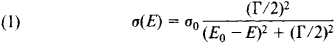The form of the maximum coincides, for example, with the relationship between the square of the amplitude of the oscillations and the frequency ω in a mechanical system when ω is varied in the vicinity of a resonance frequency. The energy E0, corresponding to the maximum of the cross section σ = σ0, is correlated with the mass M of the resonance according to the formula of the theory of relativity M = E0/c2. (In the physics of elementary particles, the mass is customarily expressed in energy units; that is, if it is assumed that c = l, then M = E0.)The quantity Γ is the total width at half maximum on the scale of energy.

The first resonance—the Δ3,3 resonance—was discovered in Chicago during the early 1950’s by E. Fermi and his colleagues while they were studying the interaction process of π+-mesons and protons on the proton cyclotron. (In modern nomenclature, the first digit in the resonance subscript indicates twice the isotopic spin I of the particle, and the second digit indicates twice the particle’s spin J. The Δ3,3 resonance can be visualized as the excited state of a nucleon (N) into which the nucleon passes after having absorbed a π-meson. The rest mass of the Δ3,3 resonance is equal to the total energy of the system N + π in the center-of-mass system of these particles: M = (1,233 ± 3) million electron volts (MeV), and the lifetime τ = 5.7 × 1024 sec. The quantity that is the inverse of τ determines the probability of the particle’s decay. In the physics of resonances, instead of the lifetime, the total energy width Γ is often used; this is related to τ by the expression τΓ ≈ , as follows from the uncertainty principle for energy and time. The resonance Δ3,3 has a total width Γ = (116 ± 6) MeV, a spin J = 3/2, and an isotopic spin I = 3/2.

In the quantum mechanical amplitude T3,3(E) of πN-scatter-ing with I = J = 3/2, this resonance appears in the form of the Breit-Wigner peak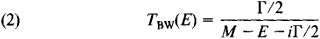for which the square of the modulus is proportional to equation (1). Here E is the total energy of the system πN in the center-of-mass system. Δ3,3 only decays into a π-meson and a nucleon. Thus, the formation and decay reactions of Δ3,3 are reciprocal: π + N ⇄ Δ3,3. Resonances that have this property are called elastic; those that may decay in two or more modes (channels) are called inelastic. A great number of resonances were discovered in the first half of the 1960’s in experiments performed with proton accelerators.

Resonances are divided into two groups: (1) baryon resonances, which have a baryon charge (B = 1) and decay into mesons and one stable baryon; and (2) meson (or boson) resonances, which decay into mesons (B = 0). Resonances with a nonzero strangeness are called strange resonances.

There are three principal methods of observing resonances: (a) by means of a maximum in the total effective scattering cross section, (b) by phase analysis, and (c) by maxima in the mass distributions.

In method (a), a bell-shaped maximum σ (E) ~ ǀTBw(E2 is observed in the total effective cross section for which the position and total width on the E scale are M and Γ, respectively. This method, however, does not permit a complete determination to be made of the quantum numbers of the resonance, particularly of the spin.

In method (b), the measured reference quantities originally measured are the cross-differential sections for elastic scattering, that is, the cross sections that are measured as functions of the scattering angle θ and the total energy E. The quantum mechanical amplitude of the scattering T(θ, E) is then expanded in a series of spherical functions, and, in the simplest spin-free case, in Legendre polynomials Pl (cos θ):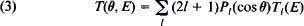The coefficients Tl (E) of this expansion, which are partial scattered waves having an angular momentum equal to the positive integer l, are determined from the experimental data in the form of complex functions of the real variable E, A resonance having a spin J = l appears in the form of a Breit-Wigner peak [equation (2)] at Tl (E). This method permits all the resonance characteristics to be determined, such as mass, width, spin, and parity.

Methods (a) and (b) are used mainly to detect baryon resonances.

Method (c) is used when processing data for inelastic reactions of the type a + b → c1 + c2 + … + cn, where η particles (n ≥ 3) result from a collision between two particles a and b. In this case, distributions are plotted for a number of events in which two or several particles have evolved in the final state, such as c1 and c2, as functions of the total energy of the particles in their center-of-mass system; in this system, the total energy E12 = E1 + E2 determines the “effective mass” M12 of the pair of particles c1 + c2. The distribution with respect to M12 is called the mass distribution. The maximum in the mass distribution around the average value M12 = M* is interpreted as a resonance having a mass M* that can decay into the particles c1 and c2. It is possible to use this method successfully in those cases where the resonance decays into a comparatively large number of particles.

A variant of this method can be termed the “deficient mass” method. It is used in those cases where, for example, n = 3, and it is easier to record the particle c3 than the particles c1 and c2. The energy of the pair of particles c1 and c2 is calculated from the difference E12 = Eab - E3 (the “deficient” energy). The resonance appears as a maximum in the distribution for the deficient mass. The method of mass distributions is the principal means of detecting meson resonances.

By November 1974, more than 200 resonances had been discovered, approximately grouped into 40 baryon and 25 meson isotopic multiplets (seeISOTOPIC INVARIANCE). The masses of the baryon resonances are between 1.2 and 3 gigaelectron volts (GeV), and the masses of the meson resonances are between 700 and 1,800 MeV. The lower limits of resonance mass spectra are determined by the masses of nuclear-stable (that is, stable with respect to decay caused by strong interactions) mesons and baryons; the upper limits depend on the experimental possibilities of detecting the resonances.

In November 1974, two new heavy meson resonances (ψ-particles) were discovered, with masses of approximately 3.1 and 3.7 GeV and unusual properties—despite the presence of meson decay, the particles ψ1 and ψ2 have a very small width (~90 kiloelectron volts and ~0.5 MeV). In January 1975, yet another meson resonance was discovered, with a mass of 4.2 GeV.

The resonances situated in the upper portion of the mass spectrum have larger spins and larger widths. The largest spin that has been determined is J = 11/2 (the resonance Δ3,11, with a mass M = 2.4 GeV). These resonances can decay in several ways. The number of possible decay channels increases rapidly with increasing energy; at energies of 1.5 to 2 GeV, baryon resonances, for example, have approximately five different decay channels. An important feature of the multiparticle decay-channel mechanism of heavy resonances is the cascade nature of the mechanism; that is, the decay occurs in many stages. Thus, for instance, the nonstrange baryon resonance Δ3,7 (I = 3/2, J = 7/2, and M = 1,950 MeV), which is produced in πN-collisions, not only has the two-particle decay Δ3,7 → π+ N, but also has other possible decays, including the predominant decay into two π-mesons and a nucleon: Δ3,7 → π + π + N. However, this process takes place in two steps: first Δ3,7 decays into a π meson and Δ3,3, and then Δ3,3 decays into a π and an N: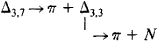In spite of some increase in the total width (that is, in the total probability of decay) with increasing energy, the probability of decay in each specific channel is decreased. This hampers the detection and study of the properties of resonances with a mass M2 GeV.

The mass spectra of resonances exhibit certain striking regularities. Thus, the resonances having the maximum spin for a given mass, parity, isotopic spin, and strangeness (ancestor resonances) are generally grouped into families of two types: (1) multiplets of a unitary-symmetry group and (2) families lying on linear Regge trajectories.

The first group, with the unitary symmetry SU(3), is a generalization of the group with the isotopic symmetry SU(2). The isotopic (or charge) symmetry reflects the fact, established experimentally, that strong interactions do not depend on electric charge. As a result, for example, a proton (p) and a neutron (n), which differ only in their electric charge (and, as a result, in their magnetic moments), participate in strong interactions in the same way and thus have very similar masses: Mp = 938.26 MeV and Mn = 939.55 MeV. The two particles form an isotopic doublet. Similarly, π+ -, π0-, and π-mesons form isotopic triplets, and so on. (The number of particles that compose one iso-topic multiplet is equal to 2I + 1.) The relative differences of the masses of the particles within isotopic multiplets are very small (<1 percent); these differences are caused by an electromagnetic interaction that disturbs the charge symmetry. Experiments have established that strong interactions are approximately independent of strangeness, and the unitary symmetry of SU (3) takes this into account.

In an approximation of unitary symmetry, nuclear-stable particles and resonances are grouped into multiplets of the unitary group SU (3). Thus, for example, nuclear-stable baryons form an octet (the nucleons η and ρ and the hyperons Σ+, Σ0, Σ-, Λ, Ξ0, and Ξ-), the Fermi resonance Δ3,3 is a member of a “decuplet” or “deket” composed of ten particles, and so on. Unitary multiplets join nuclear-stable particles and resonances having the same values of the baryon charge B, parity P, and spin J but different values of the isotopic spin I and the strangeness S. The relative differences of the masses within unitary multiplets are substantially greater than within isotopic multiplets and reach approximately 10 percent.

The concept of Regge poles was borrowed from nonrelativis-tic quantum mechanics. By a formal solution of the Schrö-dinger equation for the radial part of the wave function with complex values of the angular momentum l, it is possible to determine the generalized partial amplitude T(l, E) as a function of two continuous variables: the energy E and the complex angular momentum l. It was established by the Italian physicist T. Regge that for Yukawa-type potentials the amplitude T(l, E) has first-order poles (seeSINGULAR POINT) with respect to the variable l of the form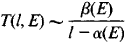where α (E) and β(E) are certain functions of the energy. These poles are called Regge poles, and the complex-valued functions α(E) are called Regge trajectories.

Since the functions T(l, E) are reduced to ordinary partial waves Tl(E) when the values of l are real positive integers (see equation ), the Regge trajectories can combine into families resonances having different values of the angular momentum. Such Regge families have been discovered in resonances. The resonances lying on a Regge trajectory have the same values for all the quantum numbers (the baryon charge, parity, strangeness, and isotopic spin) except the angular momentum l, and the spin J of the resonances is a continuous function of the resonance mass My.

(4) J = Re α(Mj)

where Re indicates the real part of the function α. Furthermore, because of certain special features of the symmetry (crossing symmetry), there are resonances on a Regge trajectory with spins that differ by 2. A typical example is the baryon trajectory αδ, which has a linear form with respect to M2:

(5) Re αδ(M) ≈ 0.1 + 0.9M2

Here, the mass M is expressed in GeV; the subscript refers to the trajectory that passes through a resonance with I = 3/2 and P = +1. Three resonances lie on this trajectory: Δ3,3(1,236), Δ3,7(1,950), and Δ3,11 (2,420). (The mass of the resonance is given in MeV in parentheses after each symbol.) Equation (5) also predicts the resonance Δ3,15 with a mass of 2,850 MeV and the resonance Δ3,19 with a mass of 3,230 MeV; the corresponding maxima in the total cross sections have been observed experimentally.

The “older” resonances, as a rule, enter into unitary multiplets and are also situated on linear (on the scale of the square of the masses) Regge trajectories. Linear trajectories have very similar slopes: α′ ≈ 0.9 GeV-2, for both baryon and meson trajectories. The linearity of Regge trajectories and the universality of the slopes have not received satisfactory theoretical explanations.

The classification of nuclear-stable particles and resonances by means of unitary multiplets and Regge trajectories demonstrates the equivalence of nuclear-stable particles and resonances. Thus, for example, the baryon decuplet mentioned previously with J = 3/2 and P = + 1 contains the resonance Δ3,3(1,236) (which includes four particles: Δ++, Δ+, Δ0, and Δ-), the resonance Σ*(1,385) (I = 1 with three particles: Σ+*, Σ0*, and Σ-*), and the resonance Ξ*(1,530) (I =½ with two particles: Ξ0* and Ξ-*), as well as the nuclear-stable hyperon Ω-(1,672) with a lifetime of 1.3 × 10-10sec.

The stable nucleon N(938) is situated on the Regge trajectory αα (the subscript α refers to a trajectory with I = ½ and P = +1):

Re αδ(M) = -0.4 + 1.0M2

together with the other resonances N*(1,690, J = 5/2), N**(2,220, J = 9/2), and so on.

Thus, the property of stability in relation to decays caused by strong interactions apparently does not have a deep physical significance and is, to some extent, the random result of relationships among the masses of the particles. In a similar way, the instability of a neutron in relation to β-decay is the result of the relationship Mn > Mp + me, where me is the mass of the electron.

The concept of the equivalence of nuclear-stable hadrons and resonances has been called nuclear democracy.

Initially, the study of resonance properties was of interest because the resonances were interpreted as the excited states (isobars) of strongly interacting elementary particles. The study of the spectra of excited atomic states has played a decisive role in the discovery of quantum mechanical regularities. Now, however, the division into “fundamental” nuclear-stable hadrons —the elementary particles—and excited states—the resonances—is at variance with the concept of nuclear democracy and is gradually becoming outmoded. The regularities of the mass spectra and of the decay properties of elementary particles that are associated with the properties of unitary symmetry have lead to the quark hypothesis. According to this hypothesis, the nuclear-stable hadrons and the hadron resonances are composed of various combinations of three hypothetical “truly elementary” particles, called quarks, and three antiquarks. In order to explain the properties of the ψ-particles, which were discovered later, it was hypothesized that there exists a fourth “charmed” quark and a corresponding antiquark. Attempts to detect quarks directly in experiments have not yet succeeded.

REFERENCES

Hill, R. D. “Rezonansnye chastitsy.” In Elementarnye chastitsy, fasc. 3. Moscow, 1965. Pages 68–82. (Translated from English.)
Dubovikov, M. S., and Iu. A. Simonov. “Raspad rezonansnykh sostoia-nii i opredelenie ikh kvantovykh chisel.” Uspekhi fizicheskikh nauk, 1970, vol. 101, issue 4, pp. 655–96.
Shirkov, D. V. “Svoistva traektorii poliusov Redzhe.” Uspekhi fizicheskikh nauk, 1970, vol. 102, issue 1, pp. 87–104.
Novozhilov, Iu. V. Vvedenie ν leoriiu elementarnykh chastils. Moscow, 1972.

D. V. SHIRKOV